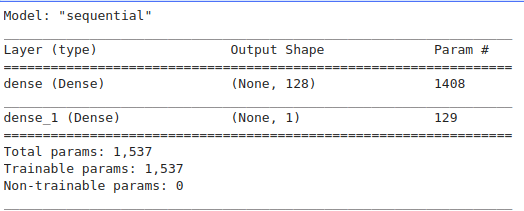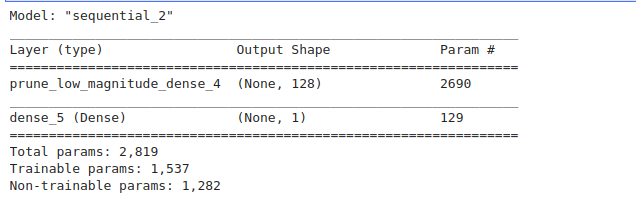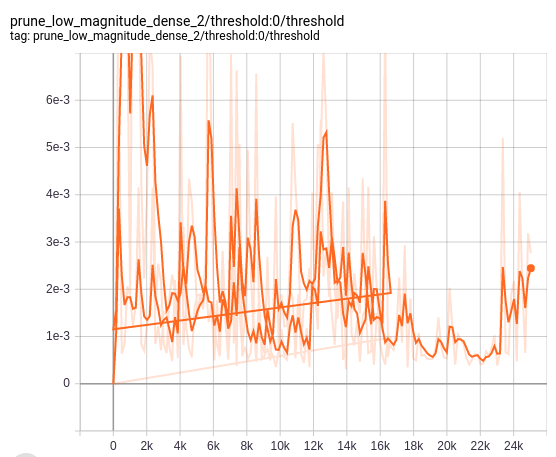## Pruning Machine Learning Models in TensorFlow

In a previous article, we reviewed some of the pre-eminent literature on pruning neural networks. We learned that pruning is a model optimization technique that involves eliminating unnecessary values in the weight tensor. This results in smaller models with accuracy very close to the baseline model.
In this article, we’ll work through an example as we apply pruning and view the effect on the final model size and prediction errors.

### Import the Usual Suspects

Our first step is to get a couple of imports out of the way:

• `Os` and `Zipfile` will help us in assessing the size of the models.
• `tensorflow_model_optimization` for model pruning.
• `load_model` for loading a saved model.
• and of course `tensorflow` and `keras`.

Finally, we initialize TensorBoard so that we’ll able to visualize the models:

``````import os
import zipfile
import tensorflow as tf
import tensorflow_model_optimization as tfmot
from tensorflow import keras

### Dataset Generation

For this experiment, we’ll generate a regression dataset using scikit-learn. Thereafter, we split the dataset into a training and test set:

``````from sklearn.datasets import make_friedman1
X, y = make_friedman1(n_samples=10000, n_features=10, random_state=0)from sklearn.model_selection import train_test_splitX_train, X_test, y_train, y_test = train_test_split(X, y, test_size=0.33, random_state=42)``````

### Model Without Pruning

We’ll create a simple neural network to predict the target variable `y`. We’ll then check the mean squared error. After this, we’ll compare this with the entire model pruned, and then with just the `Dense` layer pruned.

Next, we step up a callback to stop training the model once it stops improving, after 30 epochs.

``early_stop = keras.callbacks.EarlyStopping(monitor=’val_loss’, patience=30)``

Let’s print a summary of the model so that we can compare it with the summary of the pruned models.

``model = setup_model()model.summary()``Let’s compile the model and train it.

``````model.compile(optimizer=’adam’,
loss=tf.keras.losses.mean_squared_error,
metrics=[‘mae’, ‘mse’])model.fit(X_train,y_train,epochs=300,validation_split=0.2,callbacks=early_stop,verbose=0)``````

Since it’s a regression problem, we’re monitoring the mean absolute error and the mean squared error.

Here’s the model plotted to an image. The input is 10 since the dataset we generated has 10 features.

``````tf.keras.utils.plot_model(
model,
to_file=”model.png”,
show_shapes=True,
show_layer_names=True,
rankdir=”TB”,
expand_nested=True,
dpi=96,
)``````Let’s now check the mean squared error. We can move on to the next section and see how this error changes when we prune the entire model.

``from sklearn.metrics import mean_squared_errorpredictions = model.predict(X_test)print(‘Without Pruning MSE %.4f’ % mean_squared_error(y_test,predictions.reshape(3300,)))Without Pruning MSE 0.0201``

### Pruning the Entire Model with a ConstantSparsity Pruning Schedule

Let’s compared the above MSE with the one obtained upon pruning the entire model. The first step is to define the pruning parameters. The weight pruning is magnitude-based. This means that some weights are converted to zeros during the training process. The model becomes sparse, hence making it easier to compress. Sparse models also make inferencing faster since the zeros can be skipped.

The parameters expected are the pruning schedule, the block size, and the block pooling type.

• In this case, we’re setting a 50% sparsity, meaning that 50% of the weights will be zeroed.
• `block_size` — The dimensions (height, weight) for the block
sparse pattern in matrix weight tensors.
• `block_pooling_type` — The function to use to pool weights in the
block. Must be `AVG` or `MAX`.

We can now prune the entire model by applying our pruning parameters.

Let’s check the model summary. Compare this with the summary of the unpruned model. From the image below we can see that the entire model has been pruned—we’ll see the difference shortly with the summary obtained after pruning one dense layer.We have to compile the model before we can fit it to the training and testing set.

``````model_to_prune.compile(optimizer=’adam’,
loss=tf.keras.losses.mean_squared_error,
metrics=[‘mae’, ‘mse’])``````

Since we’re applying pruning, we have to define a couple of pruning callbacks in addition to the early stopping callback. We define the folder to log the model, then create a list with the callbacks.

`tfmot.sparsity.keras.UpdatePruningStep()` updates pruning wrappers with the optimizer step. Failure to specify it will result in an error.

`tfmot.sparsity.keras.PruningSummaries()` adds pruning summaries to the Tensorboard.

``````log_dir = ‘.models’
callbacks = [
tfmot.sparsity.keras.UpdatePruningStep(),
# Log sparsity and other metrics in Tensorboard.
tfmot.sparsity.keras.PruningSummaries(log_dir=log_dir),
keras.callbacks.EarlyStopping(monitor=’val_loss’, patience=10)
]``````

With that out of the way, we can now fit the model to the training set.

``model_to_prune.fit(X_train,y_train,epochs=100,validation_split=0.2,callbacks=callbacks,verbose=0)``

Upon checking the mean squared error for this model, we notice that it’s slightly higher than the one for the unpruned model.

``prune_predictions = model_to_prune.predict(X_test)print(‘Whole Model Pruned MSE %.4f’ % mean_squared_error(y_test,prune_predictions.reshape(3300,)))Whole Model Pruned MSE  0.1830``

### Pruning the Dense Layer Only with PolynomialDecay Pruning Schedule

Let’s now implement the same model—but this time, we’ll prune the dense layer only. Notice the use of the `PolynomialDecay` function in the pruning schedule.

From the summary, we can see that only the first dense layer will be pruned.

``model_layer_prunning.summary()``We then compile and fit the model.

``````model_layer_prunning.compile(optimizer=’adam’,
loss=tf.keras.losses.mean_squared_error,
metrics=[‘mae’, ‘mse’])model_layer_prunning.fit(X_train,y_train,epochs=300,validation_split=0.1,callbacks=callbacks,verbose=0)``````

Now, let’s check the mean squared error.

``layer_prune_predictions = model_layer_prunning.predict(X_test)print(‘Layer Prunned MSE %.4f’ % mean_squared_error(y_test,layer_prune_predictions.reshape(3300,)))Layer Prunned MSE 0.1388``

We can’t compare the MSE obtained here with the previous one since we’ve used different pruning parameters. If you’d like to compare them, then ensure that the pruning parameters are similar. Upon testing, `layer_pruning_params` gave a lower error than the `pruning_params` for this specific case. Comparing the MSE obtained from different pruning parameters is useful so that you can settle for the one that doesn’t make the model’s performance worse.

### Comparing Model Sizes

Let’s now compare the sizes of the models with and without pruning. We start by training and saving the model weights for later use.

We’ll set up our base model and load the saved weights. We then prune the entire model. We compile, fit the model, and visualize the results on Tensorboard.

Here’s a single snapshot of the pruning summaries from TensorBoard.The other pruning summaries can also be viewed on Tensorboard.Let’s now define a function to compute the sizes of the models.

And now we define the model for export and then compute the sizes.

For a pruned model,` tfmot.sparsity.keras.strip_pruning()` is used to restore the original model with the sparse weights. Notice the difference in size for the stripped and unstripped models.

``model_for_export = tfmot.sparsity.keras.strip_pruning(model_for_pruning)``
``````Size of gzipped pruned model without stripping: 6101.00 bytes
Size of gzipped pruned model with stripping: 5140.00 bytes``````

Running predictions on both models, we see that they have the same mean squared error.

``````Model for Prunning Error 0.0264
Model for Export Error  0.0264``````

### Final Thoughts

You can go ahead and test how different pruning schedules affect the size of the model. Obviously, the observations made here are not universal. You’ll have to try different pruning parameters and learn how they affect your model size, prediction error, and/or accuracy depending on your problem.

To optimize the model even more, you could quantize it. If you’d like to explore that and more, check the repo and the resources below.

### Resources

Pruning in Keras example | TensorFlow Model Optimization
Welcome to an end-to-end example for magnitude-based weight pruning. For an introduction to what pruning is and to…

Pruning comprehensive guide | TensorFlow Model Optimization
TensorFlow Lite for mobile and embedded devices

mwitiderrick/Pruning-in-TensorFlow
In this article, we comb through an example as we apply pruning and view the effect on the final model size …

Bio: Derrick Mwiti is a data scientist who has a great passion for sharing knowledge. He is an avid contributor to the data science community via blogs such as Heartbeat, Towards Data Science, Datacamp, Neptune AI, KDnuggets just to mention a few. His content has been viewed over a million times on the internet. Derrick is also an author and online instructor. He also trains and works with various institutions to implement data science solutions as well as to upskill their staff. Derrick’s studied Mathematics and Computer Science from the Multimedia University, he also is an alumnus of the Meltwater Entrepreneurial School of Technology. If the world of Data Science, Machine Learning, and Deep Learning interest you, you might want to check his Complete Data Science & Machine Learning Bootcamp in Python course.

Original. Reposted with permission.

Related: